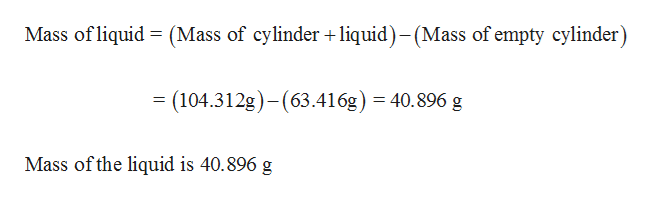# A student determines the density of a liquid by determining the mass of an empty graduated cylinder, adding 50.0mL of liquid to the cylinder and weighing the cylinder and liquid.The following data is obtained:Mass of the empty cylinder    63.416gMass of the cylinder and liquid 104.312gAnswer the following based on the preceding information. Answers should have units and be rounded to the proper number of significant figures.a) What is the mass of the liquid?b) What is the density of the liquid?

Question
18 views

A student determines the density of a liquid by determining the mass of an empty graduated cylinder, adding 50.0mL of liquid to the cylinder and weighing the cylinder and liquid.
The following data is obtained:

Mass of the empty cylinder    63.416g

Mass of the cylinder and liquid 104.312g

Answer the following based on the preceding information. Answers should have units and be rounded to the proper number of significant figures.
a) What is the mass of the liquid?

b) What is the density of the liquid?

check_circle

Step 1

(a) Determine mass o...help_outlineImage TranscriptioncloseMass of liquid (Mass of cylinder +liquid)-(Mass of empty cylinder) = (104.312g)-(63.416g) 40.896 g Mass of the liquid is 40.896 g fullscreen

### Want to see the full answer?

See Solution

#### Want to see this answer and more?

Solutions are written by subject experts who are available 24/7. Questions are typically answered within 1 hour.*

See Solution
*Response times may vary by subject and question.
Tagged in

### General Chemistry